x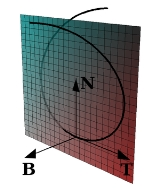Frenet-Serret formulasOverview

In vector calculus, the Frenet–Serret formulas describe the kinematic properties of a particle which moves along a continuous, differentiable curve
Curve
In mathematics, a curve is, generally speaking, an object similar to a line but which is not required to be straight...

in three-dimensional Euclidean space
Euclidean space
In mathematics, Euclidean space is the Euclidean plane and three-dimensional space of Euclidean geometry, as well as the generalizations of these notions to higher dimensions...

R3 (or the geometric properties of the curve irrespective of any motion). More specifically, the formulas describe the derivative
Derivative
In calculus, a branch of mathematics, the derivative is a measure of how a function changes as its input changes. Loosely speaking, a derivative can be thought of as how much one quantity is changing in response to changes in some other quantity; for example, the derivative of the position of a...

s of the so-called tangent, normal, and binormal unit vectors in terms of each other.Unanswered QuestionsWhat is the application of frenet serret formula in real life problem or othersEncyclopedia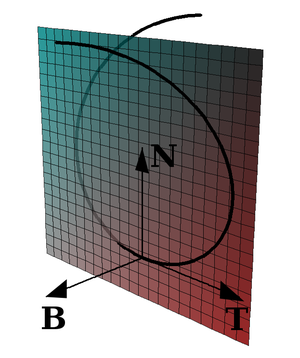In vector calculus, the Frenet–Serret formulas describe the kinematic properties of a particle which moves along a continuous, differentiable curve
Curve
In mathematics, a curve is, generally speaking, an object similar to a line but which is not required to be straight...

in three-dimensional Euclidean space
Euclidean space
In mathematics, Euclidean space is the Euclidean plane and three-dimensional space of Euclidean geometry, as well as the generalizations of these notions to higher dimensions...

R3 (or the geometric properties of the curve irrespective of any motion). More specifically, the formulas describe the derivative
Derivative
In calculus, a branch of mathematics, the derivative is a measure of how a function changes as its input changes. Loosely speaking, a derivative can be thought of as how much one quantity is changing in response to changes in some other quantity; for example, the derivative of the position of a...

s of the so-called tangent, normal, and binormal unit vectors in terms of each other. The formulas are named after the two French mathematicians who independently discovered them: Jean Frédéric Frenet
Jean Frédéric Frenet
Jean Frédéric Frenet was a French mathematician, astronomer, and meteorologist. He was born and died in Périgueux, France....

, in his thesis of 1847, and Joseph Alfred Serret
Joseph Alfred Serret
Joseph Alfred Serret was a French mathematician who was born in Paris, France, and died in Versailles, France.-Books by J. A. Serret:* * *...

in 1851. Vector notation and linear algebra currently used to write these formulas was not yet in use at the time of their discovery.

The tangent, normal, and binormal unit vectors, often called T, N, and B, or collectively the Frenet–Serret frame or TNB frame are defined as follows:
• T is the unit vector tangent
Tangent vector
A tangent vector is a vector that is tangent to a curve or surface at a given point.Tangent vectors are described in the differential geometry of curves in the context of curves in Rn. More generally, tangent vectors are elements of a tangent space of a differentiable manifold....

to the curve, pointing in the direction of motion.
• N is the derivative of T with respect to the arclength parameter of the curve, divided by its length.
• B is the cross product
Cross product
In mathematics, the cross product, vector product, or Gibbs vector product is a binary operation on two vectors in three-dimensional space. It results in a vector which is perpendicular to both of the vectors being multiplied and normal to the plane containing them...

of T and N.

The Frenet–Serret formulas arewhere d/ds is the derivative with respect to arclength, κ is the curvature
Curvature
In mathematics, curvature refers to any of a number of loosely related concepts in different areas of geometry. Intuitively, curvature is the amount by which a geometric object deviates from being flat, or straight in the case of a line, but this is defined in different ways depending on the context...

and τ is the torsion
Torsion of curves
In the elementary differential geometry of curves in three dimensions, the torsion of a curve measures how sharply it is twisting. Taken together,the curvature and the torsion of a space curve are analogous to the curvature of a plane curve...

of the curve. This formula effectively defines the curvature and torsion of a space curve.

## Frenet–Serret formulasLet r(t) be a curve
Curve
In mathematics, a curve is, generally speaking, an object similar to a line but which is not required to be straight...

in Euclidean space
Euclidean space
In mathematics, Euclidean space is the Euclidean plane and three-dimensional space of Euclidean geometry, as well as the generalizations of these notions to higher dimensions...

, representing the position vector of the particle as a function of time. The Frenet–Serret formulas apply to curves which are non-degenerate, which roughly means that they have curvature
Curvature
In mathematics, curvature refers to any of a number of loosely related concepts in different areas of geometry. Intuitively, curvature is the amount by which a geometric object deviates from being flat, or straight in the case of a line, but this is defined in different ways depending on the context...

. More formally, in this situation the velocity
Velocity
In physics, velocity is speed in a given direction. Speed describes only how fast an object is moving, whereas velocity gives both the speed and direction of the object's motion. To have a constant velocity, an object must have a constant speed and motion in a constant direction. Constant ...

vector r′(t) and the acceleration
Acceleration
In physics, acceleration is the rate of change of velocity with time. In one dimension, acceleration is the rate at which something speeds up or slows down. However, since velocity is a vector, acceleration describes the rate of change of both the magnitude and the direction of velocity. ...

vector r′′(t) are required not to be proportional.

Let s(t) represent the arc length
Arc length
Determining the length of an irregular arc segment is also called rectification of a curve. Historically, many methods were used for specific curves...

which the particle has moved along the curve
Curve
In mathematics, a curve is, generally speaking, an object similar to a line but which is not required to be straight...

. The quantity s is used to give the curve traced out by the trajectory of the particle a natural parametrization by arc length, since many different particle paths may trace out the same geometrical curve by traversing it at different rates. In detail, s is given by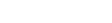Moreover, since we have assumed that r′ ≠ 0, it is possible to solve for t as a function of s, and thus to write r(s) = r(t(s)). The curve is thus parametrized in a preferred manner by its arc length.

With a non-degenerate curve r(s), parameterized by its arclength, it is now possible to define the Frenet–Serret frame (or TNB frame):
• The tangent unit vector T is defined as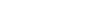• The normal unit vector N is defined as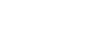• The binormal unit vector B is defined as the cross product
Cross product
In mathematics, the cross product, vector product, or Gibbs vector product is a binary operation on two vectors in three-dimensional space. It results in a vector which is perpendicular to both of the vectors being multiplied and normal to the plane containing them...

of T and N: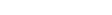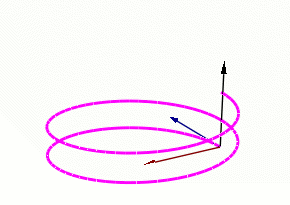From equation (2) it follows, since T always has unit magnitude
Magnitude (mathematics)
The magnitude of an object in mathematics is its size: a property by which it can be compared as larger or smaller than other objects of the same kind; in technical terms, an ordering of the class of objects to which it belongs....

, that N is always perpendicular to T. From equation (3) it follows that B is always perpendicular to both T and N. Thus, the three unit vectors T, N, and B are all perpendicular to each other.

The Frenet–Serret formulas are:whereis the curvature
Curvature
In mathematics, curvature refers to any of a number of loosely related concepts in different areas of geometry. Intuitively, curvature is the amount by which a geometric object deviates from being flat, or straight in the case of a line, but this is defined in different ways depending on the context...

andis the torsion
Torsion of curves
In the elementary differential geometry of curves in three dimensions, the torsion of a curve measures how sharply it is twisting. Taken together,the curvature and the torsion of a space curve are analogous to the curvature of a plane curve...

.

The Frenet–Serret formulas are also known as Frenet–Serret theorem, and can be stated more concisely using matrix notation: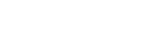This matrix is skew-symmetric
Skew-symmetric matrix
In mathematics, and in particular linear algebra, a skew-symmetric matrix is a square matrix A whose transpose is also its negative; that is, it satisfies the equation If the entry in the and is aij, i.e...

.

### Formulas in n dimensions

The Frenet–Serret formulas were generalized to higher dimensional Euclidean spaces by Camille Jordan
Camille Jordan
Marie Ennemond Camille Jordan was a French mathematician, known both for his foundational work in group theory and for his influential Cours d'analyse. He was born in Lyon and educated at the École polytechnique...

in 1874.

Suppose that r(s) is a smooth curve in Rn, parametrized by arc length, and that the first n derivatives of r are linearly independent. The vectors in the Frenet–Serret frame are an orthonormal basis
Orthonormal basis
In mathematics, particularly linear algebra, an orthonormal basis for inner product space V with finite dimension is a basis for V whose vectors are orthonormal. For example, the standard basis for a Euclidean space Rn is an orthonormal basis, where the relevant inner product is the dot product of...

constructed by applying the Gram-Schmidt process to the vectors (r′(s), r′′(s), ..., r(n)(s)).

In detail, the unit tangent vector is the first Frenet vector e1(t) and is defined as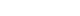The normal vector, sometimes called the curvature vector, indicates the deviance of the curve from being a straight line. It is defined as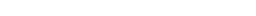Its normalized form, the unit normal vector, is the second Frenet vector e2(s) and defined as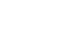The tangent and the normal vector at point s define the osculating plane
Osculating plane
In mathematics, particularly in differential geometry, an osculating plane is a plane in a Euclidean space or affine space which meets a submanifold at a point in such a way as to have a second order of contact at the point. The word osculate is from the Latin osculatus which is a past participle...

at point r(s).

The remaining vectors in the frame (the binormal, trinormal, etc.) are defined similarly by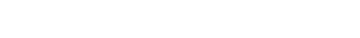The real valued functions χi(s) are called generalized curvature and are defined as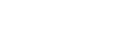The Frenet–Serret formulas, stated in matrix language, are## Proof

Consider the matrix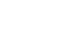The rows of this matrix are mutually perpendicular unit vectors: an orthonormal basis
Orthonormal basis
In mathematics, particularly linear algebra, an orthonormal basis for inner product space V with finite dimension is a basis for V whose vectors are orthonormal. For example, the standard basis for a Euclidean space Rn is an orthonormal basis, where the relevant inner product is the dot product of...

of R3. As a result, the transpose of Q is equal to the inverse of Q: Q is an orthogonal matrix
Orthogonal matrix
In linear algebra, an orthogonal matrix , is a square matrix with real entries whose columns and rows are orthogonal unit vectors ....

. It suffices to show that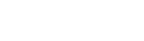Note the first row of this equation already holds, by definition of the normal N and curvature κ. So it suffices to show that (dQ/ds)QT is a skew-symmetric matrix. Since I = QQT, taking a derivative and applying the product rule yields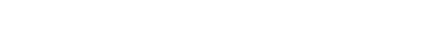which establishes the required skew-symmetry.

### Kinematics of the frame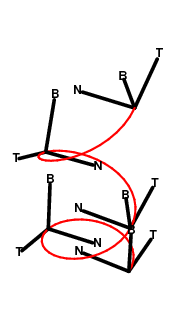The Frenet–Serret frame consisting of the tangent T, normal N, and binormal B collectively forms an orthonormal basis
Orthonormal basis
In mathematics, particularly linear algebra, an orthonormal basis for inner product space V with finite dimension is a basis for V whose vectors are orthonormal. For example, the standard basis for a Euclidean space Rn is an orthonormal basis, where the relevant inner product is the dot product of...

of 3-space. At each point of the curve, this attaches a reference frame
Reference frame
Reference frame may refer to:*Frame of reference, in physics*Reference frame , frames of a compressed video that are used to define future frames...

or rectilinear
Rectilinear
Rectilinear may refer to:* Rectilinear grid, a tessellation of the Euclidean plane* Rectilinear lens, a photographic lens* Rectilinear locomotion, a form of animal locomotion* Rectilinear polygon, a polygon whose edges meet at right angles...

coordinate system
Coordinate system
In geometry, a coordinate system is a system which uses one or more numbers, or coordinates, to uniquely determine the position of a point or other geometric element. The order of the coordinates is significant and they are sometimes identified by their position in an ordered tuple and sometimes by...

(see image).

The Frenet–Serret formulas admit a kinematic
Kinematics
Kinematics is the branch of classical mechanics that describes the motion of bodies and systems without consideration of the forces that cause the motion....

interpretation. Imagine that an observer moves along the curve in time, using the attached frame at each point as her coordinate system. The Frenet–Serret formulas mean that this coordinate system is constantly rotating as an observer moves along the curve. Hence, this coordinate system is always non-inertial
Non-inertial reference frame
A non-inertial reference frame is a frame of reference that is under acceleration. The laws of physics in such a frame do not take on their most simple form, as required by the theory of special relativity...

. The angular momentum
Angular momentum
In physics, angular momentum, moment of momentum, or rotational momentum is a conserved vector quantity that can be used to describe the overall state of a physical system...

of the observer's coordinate system is proportional to the Darboux vector
Darboux vector
In differential geometry, especially the theory of space curves, the Darboux vector is the areal velocity vector of the Frenet frame of a space curve. It is named after Gaston Darboux who discovered it...

of the frame.Concretely, suppose that the observer carries an (inertial) top
Top
A top is a toy that can be spun on an axis, balancing on a point. This motion is produced in the most simple forms of top by twirling the stem using the fingers. More sophisticated tops are spun by by holding the axis firmly while pulling a string or twisting a stick or pushing an auger as shown...

(or gyroscope
Gyroscope
A gyroscope is a device for measuring or maintaining orientation, based on the principles of angular momentum. In essence, a mechanical gyroscope is a spinning wheel or disk whose axle is free to take any orientation...

) with herself along the curve. If the axis of the top points along the tangent to the curve, then it will be observed to rotate about its axis with angular velocity -τ relative to the observer's non-inertial coordinate system. If, on the other hand, the axis of the top points in the binormal direction, then it is observed to rotate with angular velocity -κ. This is easily visualized in the case when the curvature is a positive constant and the torsion vanishes. The observer is then in uniform circular motion
Uniform circular motion
In physics, uniform circular motion describes the motion of a body traversing a circular path at constant speed. The distance of the body from the axis of rotation remains constant at all times. Though the body's speed is constant, its velocity is not constant: velocity, a vector quantity, depends...

. If the top points in the direction of the binormal, then by conservation of angular momentum it must rotate in the opposite direction of the circular motion. In the limiting case when the curvature vanishes, the observer's normal precesses about the tangent vector, and similarly the top will rotate in the opposite direction of this precession.

Applications. The kinematics of the frame have many applications in the sciences.
• In the life sciences
Life sciences
The life sciences comprise the fields of science that involve the scientific study of living organisms, like plants, animals, and human beings. While biology remains the centerpiece of the life sciences, technological advances in molecular biology and biotechnology have led to a burgeoning of...

, particularly in models of microbial motion, considerations of the Frenet-Serret frame have been used to explain the mechanism by which a moving organism in a viscous medium changes its direction.
• In physics, the Frenet-Serret frame is useful when it is impossible or inconvenient to assign a natural coordinate system for a trajectory. Such is often the case, for instance, in relativity theory. Within this setting, Frenet-Serret frames have been used to model the precession of a gyroscope in a gravitational well.

### Frenet–Serret formulas in calculus

The Frenet–Serret formulas are frequently introduced in courses on multivariable calculus
Multivariable calculus
Multivariable calculus is the extension of calculus in one variable to calculus in more than one variable: the differentiated and integrated functions involve multiple variables, rather than just one....

as a companion to the study of space curves such as the helix
Helix
A helix is a type of smooth space curve, i.e. a curve in three-dimensional space. It has the property that the tangent line at any point makes a constant angle with a fixed line called the axis. Examples of helixes are coil springs and the handrails of spiral staircases. A "filled-in" helix – for...

. A helix can be characterized by the height h and radius r of a single turn. The curvature and torsion of a helix (with constant radius) are given by the formulas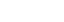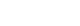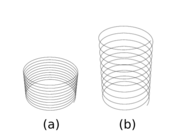The sign of the torsion is determined by the right-handed or left-handed sense
Right-hand rule
In mathematics and physics, the right-hand rule is a common mnemonic for understanding notation conventions for vectors in 3 dimensions. It was invented for use in electromagnetism by British physicist John Ambrose Fleming in the late 19th century....

in which the helix twists around its central axis. Explicitly, the parametrization of a single turn of a right-handed helix with height 2πh and radius r is
x = r cos t
y = r sin t
z = h t
(0 ≤ t ≤ 2 π)

and, for a left-handed helix,
x = r cos t
y = −r sin t
z = h t
(0 ≤ t ≤ 2 π).

Note that these are not the arc length parametrizations (in which case, each of x, y, and z would need to be divided by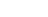.)

In his expository writings on the geometry of curves, Rudy Rucker
Rudy Rucker
Rudolf von Bitter Rucker is an American mathematician, computer scientist, science fiction author, and philosopher, and is one of the founders of the cyberpunk literary movement. The author of both fiction and non-fiction, he is best known for the novels in the Ware Tetralogy, the first two of...

employs the model of a slinky
Slinky
Slinky or "Lazy Spring" is a toy consisting of a helical spring that stretches and can bounce up and down. It can perform a number of tricks, including traveling down a flight of steps end-over-end as it stretches and re-forms itself with the aid of gravity and its own momentum.-History:The toy was...

to explain the meaning of the torsion and curvature. The slinky, he says, is characterized by the property that the quantity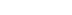remains constant if the slinky is vertically stretched out along its central axis. (Here 2πh is the height of a single twist of the slinky, and r the radius.) In particular, curvature and torsion are complementary in the sense that the torsion can be increased at the expense of curvature by stretching out the slinky.

### Taylor expansion

Repeatedly differentiating the curve and applying the Frenet–Serret formulas gives the following Taylor approximation
Taylor's theorem
In calculus, Taylor's theorem gives an approximation of a k times differentiable function around a given point by a k-th order Taylor-polynomial. For analytic functions the Taylor polynomials at a given point are finite order truncations of its Taylor's series, which completely determines the...

to the curve near s = 0: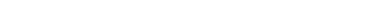For a generic curve with nonvanishing torsion, the projection of the curve onto various coordinate planes in the T, N, B coordinate system at have the following interpretations:
• The osculating plane
Osculating plane
In mathematics, particularly in differential geometry, an osculating plane is a plane in a Euclidean space or affine space which meets a submanifold at a point in such a way as to have a second order of contact at the point. The word osculate is from the Latin osculatus which is a past participle...

is the plane containing
Linear span
In the mathematical subfield of linear algebra, the linear span of a set of vectors in a vector space is the intersection of all subspaces containing that set...

T and N. The projection of the curve onto this plane has the form: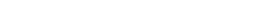This is a parabola
Parabola
In mathematics, the parabola is a conic section, the intersection of a right circular conical surface and a plane parallel to a generating straight line of that surface...

up to terms of order o(s2), whose curvature at 0 is equal to κ(0).

• The normal plane
Normal plane
A normal plane may refer to*The plane perpendicular to the tangent vector of a space curve; see Frenet–Serret formulas.*A term involving gears; see list of gear nomenclature....

is the plane containing N and B. The projection of the curve onto this plane has the form: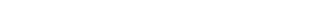which is a cuspidal cubic to order o(s3).

• The rectifying plane is the plane containing T and B. The projection of the curve onto this plane is: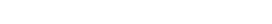which traces out the graph of a cubic polynomial to order o(s3).

### Ribbons and tubes

The Frenet–Serret apparatus allows one to define certain optimal ribbons and tubes centered around a curve. These have diverse applications in materials science
Materials science
Materials science is an interdisciplinary field applying the properties of matter to various areas of science and engineering. This scientific field investigates the relationship between the structure of materials at atomic or molecular scales and their macroscopic properties. It incorporates...

and elasticity theory, as well as to computer graphics
Computer graphics
Computer graphics are graphics created using computers and, more generally, the representation and manipulation of image data by a computer with help from specialized software and hardware....

.

A Frenet ribbon along a curve C is the surface traced out by sweeping the line segment [−N,N] generated by the unit normal along the curve. Geometrically, a ribbon is a piece of the envelope
Envelope (mathematics)
In geometry, an envelope of a family of curves in the plane is a curve that is tangent to each member of the family at some point. Classically, a point on the envelope can be thought of as the intersection of two "adjacent" curves, meaning the limit of intersections of nearby curves...

of the osculating planes of the curve. Symbolically, the ribbon R has the following parametrization: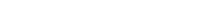In particular, the binormal B is a unit vector normal to the ribbon. Moreover, the ribbon is a ruled surface
Ruled surface
In geometry, a surface S is ruled if through every point of S there is a straight line that lies on S. The most familiar examples are the plane and the curved surface of a cylinder or cone...

whose reguli are the line segments spanned by N. Thus each of the frame vectors T, N, and B can be visualized entirely in terms of the Frenet ribbon.

The Gauss curvature of a Frenet ribbon vanishes, and so it is a developable surface
Developable surface
In mathematics, a developable surface is a surface with zero Gaussian curvature. That is, it is a "surface" that can be flattened onto a plane without distortion . Conversely, it is a surface which can be made by transforming a plane...

. Geometrically, it is possible to "roll" a plane along the ribbon without slipping or twisting so that the regulus always remains within the plane. The ribbon then traces out a ribbon in the plane (possibly with multiple sheets). The curve C also traces out a curve CP in the plane, whose curvature is given in terms of the curvature and torsion of C by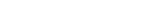This fact gives a general procedure for constructing any Frenet ribbon. Intuitively, one can cut out a curved ribbon from a flat piece of paper. Then by bending the ribbon out into space without tearing it, one produces a Frenet ribbon. In the simple case of the slinky, the ribbon is several turns of an annulus
Annulus (mathematics)
In mathematics, an annulus is a ring-shaped geometric figure, or more generally, a term used to name a ring-shaped object. Or, it is the area between two concentric circles...

in the plane, and bending it up into space corresponds to stretching out the slinky.

### Congruence of curves

In classical Euclidean geometry
Euclidean geometry
Euclidean geometry is a mathematical system attributed to the Alexandrian Greek mathematician Euclid, which he described in his textbook on geometry: the Elements. Euclid's method consists in assuming a small set of intuitively appealing axioms, and deducing many other propositions from these...

, one is interested in studying the properties of figures in the plane which are invariant under congruence, so that if two figures are congruent then they must have the same properties. The Frenet-Serret apparatus presents the curvature and torsion as numerical invariants of a space curve.

Roughly speaking, two curves C and C′ in space are congruent if one can be rigidly moved to the other. A rigid motion consists of a combination of a translation and a rotation. A translation moves one point of C to a point of C′. The rotation then adjusts the orientation of the curve C to line up with that of C′. Such a combination of translation and rotation is called a Euclidean motion. In terms of the parametrization r(t) defining the first curve C, a general Euclidean motion of C is a composite of the following operations:
• (Translation.) r(t) → r(t) + v, where v is a constant vector.
• (Rotation.) r(t) + v → M(r(t) + v), where M is the matrix of a rotation.

The Frenet–Serret frame is particularly well-behaved with regard to Euclidean motions. First, since T, N, and B can all be given as successive derivatives of the parametrization of the curve, each of them is insensitive to the addition of a constant vector to r(t). Intuitively, the TNB frame attached to r(t) is the same as the TNB frame attached to the new curve r(t) + v.

This leaves only the rotations to consider. Intuitively, if we apply a rotation M to the curve, then the TNB frame also rotates. More precisely, the matrix Q whose rows are the TNB vectors of the Frenet-Serret frame changes by the matrix of a rotation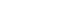A fortiori, the matrix (dQ/ds)QT is unaffected by a rotation: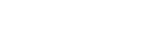since MMT = I for the matrix of a rotation.

Hence the entries κ and τ of (dQ/ds)QT are invariants of the curve under Euclidean motions: if a Euclidean motion is applied to a curve, then the resulting curve has the same curvature and torsion.

Moreover, using the Frenet–Serret frame, one can also prove the converse: any two curves having the same curvature and torsion functions must be congruent by a Euclidean motion. Roughly speaking, the Frenet–Serret formulas express the Darboux derivative
Darboux derivative
The Darboux derivative of a map between a manifold and a Lie group is a variant of the standard derivative. In a certain sense, it is arguably a more natural generalization of the single-variable derivative...

of the TNB frame. If the Darboux derivatives of two frames are equal, then a version of the fundamental theorem of calculus
Fundamental theorem of calculus
The first part of the theorem, sometimes called the first fundamental theorem of calculus, shows that an indefinite integration can be reversed by a differentiation...

asserts that the curves are congruent. In particular, the curvature and torsion are a complete set of invariants for a curve in three-dimensions.

## Other expressions of the frame

The formulas given above for T, N, and B depend on the curve being given in terms of the arclength parameter. This is a natural assumption in Euclidean geometry, because the arclength is a Euclidean invariant of the curve. In the terminology of physics, the arclength parametrization is a natural choice of gauge
Gauge theory
In physics, gauge invariance is the property of a field theory in which different configurations of the underlying fundamental but unobservable fields result in identical observable quantities. A theory with such a property is called a gauge theory...

. However, it may be awkward to work with in practice. A number of other equivalent expressions are available.

Suppose that the curve is given by r(t), where the parameter t need no longer be arclength. Then the unit tangent vector T may be written as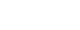The normal vector N takes the form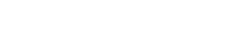The binormal B is then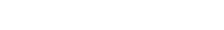An alternative way to arrive at the same expressions is to take the first three derivatives of the curve r′(t), r′′(t), r′′′(t), and to apply the Gram-Schmidt process. The resulting ordered orthonormal basis
Orthonormal basis
In mathematics, particularly linear algebra, an orthonormal basis for inner product space V with finite dimension is a basis for V whose vectors are orthonormal. For example, the standard basis for a Euclidean space Rn is an orthonormal basis, where the relevant inner product is the dot product of...

is precisely the TNB frame. This procedure also generalizes to produce Frenet frames in higher dimensions.

In terms of the parameter t, the Frenet–Serret formulas pick up an additional factor of ||r′(t)|| because of the chain rule
Chain rule
In calculus, the chain rule is a formula for computing the derivative of the composition of two or more functions. That is, if f is a function and g is a function, then the chain rule expresses the derivative of the composite function in terms of the derivatives of f and g.In integration, the...

: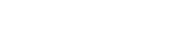## Special cases

If the curvature is always zero then the curve will be a straight line. Here the vectors N, B and the torsion are not well defined.

If the torsion is always zero then the curve will lie in a plane. A circle
Circle
A circle is a simple shape of Euclidean geometry consisting of those points in a plane that are a given distance from a given point, the centre. The distance between any of the points and the centre is called the radius....

of radius r has zero torsion and curvature equal to 1/r.

A helix
Helix
A helix is a type of smooth space curve, i.e. a curve in three-dimensional space. It has the property that the tangent line at any point makes a constant angle with a fixed line called the axis. Examples of helixes are coil springs and the handrails of spiral staircases. A "filled-in" helix – for...

has constant curvature and constant torsion.

## See also

• Affine geometry of curves
• Differential geometry of curves
Differential geometry of curves
Differential geometry of curves is the branch of geometry that dealswith smooth curves in the plane and in the Euclidean space by methods of differential and integral calculus....

• Darboux frame
Darboux frame
In the differential geometry of surfaces, a Darboux frame is a natural moving frame constructed on a surface. It is the analog of the Frenet–Serret frame as applied to surface geometry. A Darboux frame exists at any non-umbilic point of a surface embedded in Euclidean space...

• Kinematics
Kinematics
Kinematics is the branch of classical mechanics that describes the motion of bodies and systems without consideration of the forces that cause the motion....

• Moving frame
Moving frame
In mathematics, a moving frame is a flexible generalization of the notion of an ordered basis of a vector space often used to study the extrinsic differential geometry of smooth manifolds embedded in a homogeneous space.-Introduction:...

## External links

The source of this article is wikipedia, the free encyclopedia.  The text of this article is licensed under the GFDL.